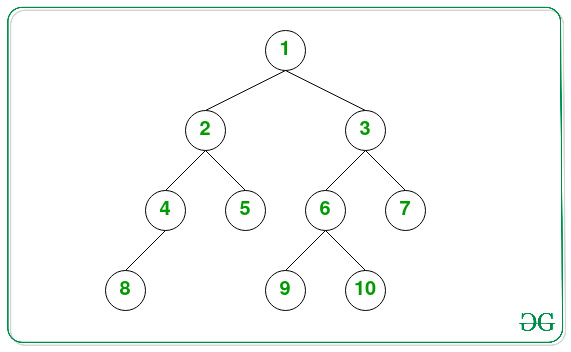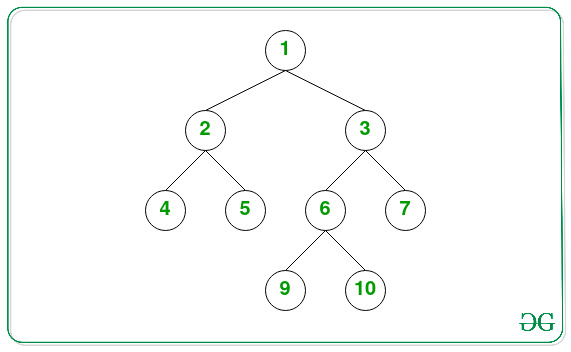Skip to content
Related Articles
Lowest Common Ancestor of the deepest leaves of a Binary Tree
• Difficulty Level : Medium
• Last Updated : 18 Jun, 2021

Given a Binary Tree consisting of N nodes having distinct values from the range [1, N], the task is to find the lowest common ancestor of the deepest leaves of the binary tree.

Examples:

Input:Output: 1
Explanation: The deepest leaf nodes of the tree are {8, 9, 10}. Lowest common ancestor of these nodes is 1.

Input:Output: 6

Approach: The given problem can be solved by finding the maximum depth of the tree and then perform the DFS Traversal to find the lowest common ancestor. Follow the steps below to solve the problem:

• Find the maximum depth of a binary tree and store it in a variable, say depth.
• Declare a function say DFS(root, curr) to find the LCS of nodes at the level depth:
• If the root is NULL, then return NULL.
• If the value of curr is equal to depth, then return the current node.
• Recursively call to the left subtree as DFS(root⇾left, curr + 1) and store the returned value in a variable say left.
• Recursively call to the right subtree as DFS(root⇾right, curr + 1) and store the returned value in a variable say right.
• If the value of the left and the right both are NOT NULL, then return the current node as the current node is the lowest common ancestor.
• If the left is NOT NULL, then return left. Otherwise, return right.
• After completing the above steps, print the value returned by the function call DFS(root, 0).

Below is the implementation of the above approach:

## C++

 `// C++ program for the above approach` `#include ``using` `namespace` `std;` `// Node of a Binary Tree``struct` `Node {``    ``struct` `Node* left;``    ``struct` `Node* right;``    ``int` `data;``};` `// Function to create``// a new tree Node``Node* newNode(``int` `key)``{``    ``Node* temp = ``new` `Node;``    ``temp->data = key;``    ``temp->left = temp->right = NULL;``    ``return` `temp;``}` `// Function to find the depth``// of the Binary Tree``int` `finddepth(Node* root)``{``    ``// If root is not null``    ``if` `(!root)``        ``return` `0;` `    ``// Left recursive subtree``    ``int` `left = finddepth(root->left);` `    ``// Right recursive subtree``    ``int` `right = finddepth(root->right);` `    ``// Returns the maximum depth``    ``return` `1 + max(left, right);``}` `// Function to perform the depth``// first search on the binary tree``Node* dfs(Node* root, ``int` `curr,``          ``int` `depth)``{``    ``// If root is null``    ``if` `(!root)``        ``return` `NULL;` `    ``// If curr is equal to depth``    ``if` `(curr == depth)``        ``return` `root;` `    ``// Left recursive subtree``    ``Node* left = dfs(root->left,``                     ``curr + 1, depth);` `    ``// Right recursive subtree``    ``Node* right = dfs(root->right,``                      ``curr + 1, depth);` `    ``// If left and right are not null``    ``if` `(left != NULL && right != NULL)``        ``return` `root;` `    ``// Return left, if left is not null``    ``// Otherwise return right``    ``return` `left ? left : right;``}` `// Function to find the LCA of the``// deepest nodes of the binary tree``Node* lcaOfDeepestLeaves(Node* root)``{``    ``// If root is null``    ``if` `(!root)``        ``return` `NULL;` `    ``// Stores the deepest depth``    ``// of the binary tree``    ``int` `depth = finddepth(root) - 1;` `    ``// Return the LCA of the``    ``// nodes at level depth``    ``return` `dfs(root, 0, depth);``}` `// Driver Code``int` `main()``{``    ``// Given Binary Tree``    ``Node* root = newNode(1);``    ``root->left = newNode(2);``    ``root->right = newNode(3);``    ``root->left->left = newNode(4);``    ``root->left->right = newNode(5);``    ``root->right->left = newNode(6);``    ``root->right->right = newNode(7);``    ``root->right->left->left = newNode(8);``    ``root->right->left->right = newNode(9);` `    ``cout << lcaOfDeepestLeaves(root)->data;` `    ``return` `0;``}`

## Java

 `// Java program for the above approach` `// Node of a Binary Tree``class` `Node``{``    ``Node left = ``null``;``    ``Node right = ``null``;``    ``int` `data;``    ` `    ``Node(``int` `data)``    ``{``        ``this``.data = data;``    ``}``}` `class` `GFG{` `// Function to find the depth``// of the Binary Tree``public` `static` `int` `findDepth(Node root)``{``    ` `    ``// If root is not null``    ``if` `(root == ``null``)``        ``return` `0``;` `    ``// Left recursive subtree``    ``int` `left = findDepth(root.left);` `    ``// Right recursive subtree``    ``int` `right = findDepth(root.right);` `    ``// Returns the maximum depth``    ``return` `1` `+ Math.max(left, right);``}` `// Function to perform the depth``// first search on the binary tree``public` `static` `Node DFS(Node root, ``int` `curr,``                                  ``int` `depth)``{``    ` `    ``// If root is null``    ``if` `(root == ``null``)``        ``return` `null``;` `    ``// If curr is equal to depth``    ``if` `(curr == depth)``        ``return` `root;` `    ``// Left recursive subtree``    ``Node left = DFS(root.left, curr + ``1``, depth);` `    ``// Right recursive subtree``    ``Node right = DFS(root.right, curr + ``1``, depth);` `    ``// If left and right are not null``    ``if` `(left != ``null` `&& right != ``null``)``        ``return` `root;` `    ``// Return left, if left is not null``    ``// Otherwise return right``    ``return` `(left != ``null``) ? left : right;``}` `// Function to find the LCA of the``// deepest nodes of the binary tree``public` `static` `Node lcaOfDeepestLeaves(Node root)``{``    ` `    ``// If root is null``    ``if` `(root == ``null``)``        ``return` `null``;` `    ``// Stores the deepest depth``    ``// of the binary tree``    ``int` `depth = findDepth(root) - ``1``;` `    ``// Return the LCA of the``    ``// nodes at level depth``    ``return` `DFS(root, ``0``, depth);``}` `// Driver code``public` `static` `void` `main(String[] args)``{``    ` `    ``// Given Binary Tree``    ``Node root = ``new` `Node(``1``);``    ``root.left = ``new` `Node(``2``);``    ``root.right = ``new` `Node(``3``);``    ``root.left.left = ``new` `Node(``4``);``    ``root.left.right = ``new` `Node(``5``);``    ``root.right.left = ``new` `Node(``6``);``    ``root.right.right = ``new` `Node(``7``);``    ``root.right.left.left = ``new` `Node(``8``);``    ``root.right.left.right = ``new` `Node(``9``);` `    ``System.out.println(lcaOfDeepestLeaves(root).data);``}``}` `// This code is contributed by girishthatte`

## Python3

 `# Python3 program for the above approach` `# Node of a Binary Tree``class` `Node:``    ``def` `__init__(``self``, d):``        ``self``.data ``=` `d``        ``self``.left ``=` `None``        ``self``.right ``=` `None` `# Function to find the depth``# of the Binary Tree``def` `finddepth(root):``    ``# If root is not null``    ``if` `(``not` `root):``        ``return` `0` `    ``# Left recursive subtree``    ``left ``=` `finddepth(root.left)` `    ``# Right recursive subtree``    ``right ``=` `finddepth(root.right)` `    ``# Returns the maximum depth``    ``return` `1` `+` `max``(left, right)` `# Function to perform the depth``# first search on the binary tree``def` `dfs(root, curr, depth):``    ``# If root is null``    ``if` `(``not` `root):``        ``return` `None` `    ``# If curr is equal to depth``    ``if` `(curr ``=``=` `depth):``        ``return` `root` `    ``# Left recursive subtree``    ``left ``=` `dfs(root.left, curr ``+` `1``, depth)` `    ``# Right recursive subtree``    ``right ``=` `dfs(root.right, curr ``+` `1``, depth)` `    ``# If left and right are not null``    ``if` `(left !``=` `None` `and` `right !``=` `None``):``        ``return` `root` `    ``# Return left, if left is not null``    ``# Otherwise return right``    ``return` `left ``if` `left ``else` `right` `# Function to find the LCA of the``# deepest nodes of the binary tree``def` `lcaOfDeepestLeaves(root):``  ` `    ``# If root is null``    ``if` `(``not` `root):``        ``return` `None` `    ``# Stores the deepest depth``    ``# of the binary tree``    ``depth ``=` `finddepth(root) ``-` `1` `    ``# Return the LCA of the``    ``# nodes at level depth``    ``return` `dfs(root, ``0``, depth)` `# Driver Code``if` `__name__ ``=``=` `'__main__'``:``  ` `    ``# Given Binary Tree``    ``root ``=` `Node(``1``)``    ``root.left ``=` `Node(``2``)``    ``root.right ``=` `Node(``3``)``    ``root.left.left ``=` `Node(``4``)``    ``root.left.right ``=` `Node(``5``)``    ``root.right.left ``=` `Node(``6``)``    ``root.right.right ``=` `Node(``7``)``    ``root.right.left.left ``=` `Node(``8``)``    ``root.right.left.right ``=` `Node(``9``)` `    ``print``(lcaOfDeepestLeaves(root).data)` `# This code is contributed by mohit kumar 29.`

## C#

 `// C# program for the above approach``using` `System;` `// Node of a Binary Tree``class` `Node``{``    ``public` `Node left = ``null``;``    ``public` `Node right = ``null``;``    ``public` `int` `data;``    ` `    ``public` `Node(``int` `data)``    ``{``        ``this``.data = data;``    ``}``}` `public` `class` `GFG{` `// Function to find the depth``// of the Binary Tree``static` `int` `findDepth(Node root)``{``    ` `    ``// If root is not null``    ``if` `(root == ``null``)``        ``return` `0;` `    ``// Left recursive subtree``    ``int` `left = findDepth(root.left);` `    ``// Right recursive subtree``    ``int` `right = findDepth(root.right);` `    ``// Returns the maximum depth``    ``return` `1 + Math.Max(left, right);``}` `// Function to perform the depth``// first search on the binary tree``static` `Node DFS(Node root, ``int` `curr,``                           ``int` `depth)``{``    ` `    ``// If root is null``    ``if` `(root == ``null``)``        ``return` `null``;` `    ``// If curr is equal to depth``    ``if` `(curr == depth)``        ``return` `root;` `    ``// Left recursive subtree``    ``Node left = DFS(root.left, curr + 1, depth);` `    ``// Right recursive subtree``    ``Node right = DFS(root.right, curr + 1, depth);` `    ``// If left and right are not null``    ``if` `(left != ``null` `&& right != ``null``)``        ``return` `root;` `    ``// Return left, if left is not null``    ``// Otherwise return right``    ``return` `(left != ``null``) ? left : right;``}` `// Function to find the LCA of the``// deepest nodes of the binary tree``static` `Node lcaOfDeepestLeaves(Node root)``{``    ` `    ``// If root is null``    ``if` `(root == ``null``)``        ``return` `null``;` `    ``// Stores the deepest depth``    ``// of the binary tree``    ``int` `depth = findDepth(root) - 1;` `    ``// Return the LCA of the``    ``// nodes at level depth``    ``return` `DFS(root, 0, depth);``}` `// Driver code``public` `static` `void` `Main(String[] args)``{``    ` `    ``// Given Binary Tree``    ``Node root = ``new` `Node(1);``    ``root.left = ``new` `Node(2);``    ``root.right = ``new` `Node(3);``    ``root.left.left = ``new` `Node(4);``    ``root.left.right = ``new` `Node(5);``    ``root.right.left = ``new` `Node(6);``    ``root.right.right = ``new` `Node(7);``    ``root.right.left.left = ``new` `Node(8);``    ``root.right.left.right = ``new` `Node(9);` `    ``Console.WriteLine(lcaOfDeepestLeaves(root).data);``}``}` `// This code is contributed by 29AjayKumar`

## Javascript

 ``
Output:
`6`

Time Complexity: O(N)
Auxiliary Space: O(1)

Attention reader! Don’t stop learning now. Get hold of all the important DSA concepts with the DSA Self Paced Course at a student-friendly price and become industry ready.  To complete your preparation from learning a language to DS Algo and many more,  please refer Complete Interview Preparation Course.

In case you wish to attend live classes with industry experts, please refer Geeks Classes Live

My Personal Notes arrow_drop_up